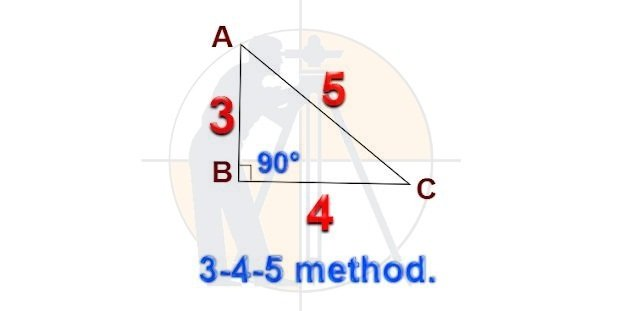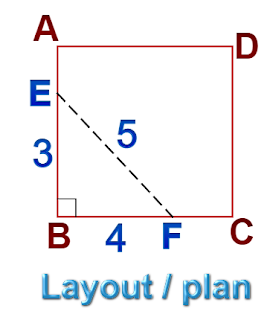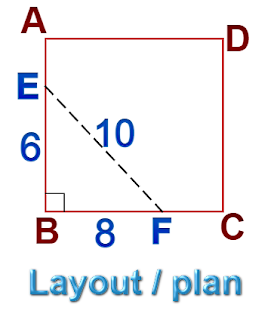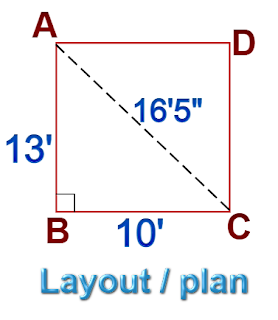Breaking News
Home / Civil Engineering / What is the 3-4-5 rule or the 3-4-5 method In Building Work

# What is the 3-4-5 rule or the 3-4-5 method In Building Work

## What is the 3-4-5 rule or the 3-4-5 method In Building Work

### 1. What is the 3-4-5 rule or the 3-4-5 method?

According to the Pythagoras theorem, in a right angle triangle,

c2=a2+ b2

Where a,b, & c are the sides of the triangleSuppose, if the side AB = 3ft. & side BC = 4ft. then,

Side AC2=AB2+ BC2

i.e. AC = √AB2+ BC2

AC = √32+ 42

AC = √9 + 16

AC = √25 = 5ft.

3. ### Estimating Of Steel Bar Quantity for Construction

Now, let us observe how we can use this method to do a layout in construction work.

### 2. How to do a layout of the building plan with a 3-4-5 rule?

#### Let us consider a building ABCD, having four walls, i.e. AB, BC, CD, & DA.To make the junction point B of the walls at a right angle, let us measure & mark 3ft. on side AB & 4ft. on side BC before constructing these walls.

Now, we have to adjust the alignment of these sides until we get the length of EF as 5ft.

This procedure makes the wall corners of the building at a right angle to each other.

A similar step has to be followed to all 4 walls of the building, to make their corners at a right angle to each other.

### 3. Where we can use the 3-4-5 rule in the construction works?

This 3-4-5 rule can be applied to do the layout of all types of structures like residential buildings, underground tanks, outhouses, etc. If the corner of the site or plot is perpendicular to each other, you can use this method to complete the layout work. All you need is a measuring tape & a lineout string.

### 4. What should be the unit of the 3-4-5 method?

You can use any unit of measurement in this 3-4-5 rule. Suppose, if you measure the sides in 3m & 4m length, the hypotenuse should be measured for 5m. length. Similarly for 3ft, & 4ft, measurement, the hypotenuse becomes 5ft.

### 5. Can we measure the length in multiples of the 3-4-5 method?

Yes. As you can observe in the drawing, if you measure 6ft. & 8ft. along the sides, the hypotenuse should be measured for 10ft. length.Similarly, by multiplying 3-4-5 by 3, we can use the measurement 9 -12-15 to carry the layout work.

### 6. Can we take any other measurements to make the corners at right angles?

Yes. As you can observe in the drawing, the two sides of a building are 10ft. & 13ft. in length.### Now, AC2=AB2+ BC2

AC = √AB2+ BC2

AC = √132+ 102

AC = √169+ 100

AC = √269 =16′ 5″

Here, you have to calculate the hypotenuse for any convenient length you have taken. By calculating the diagonal of building ABCD, you can fix all the corners at right angles easily.

Here, AC=BD=16’5″

By fixing the intersection points of the building sides & diagonals at A, B, C, &D, the building can be set in a truly rectangular shape.

### THANKS.